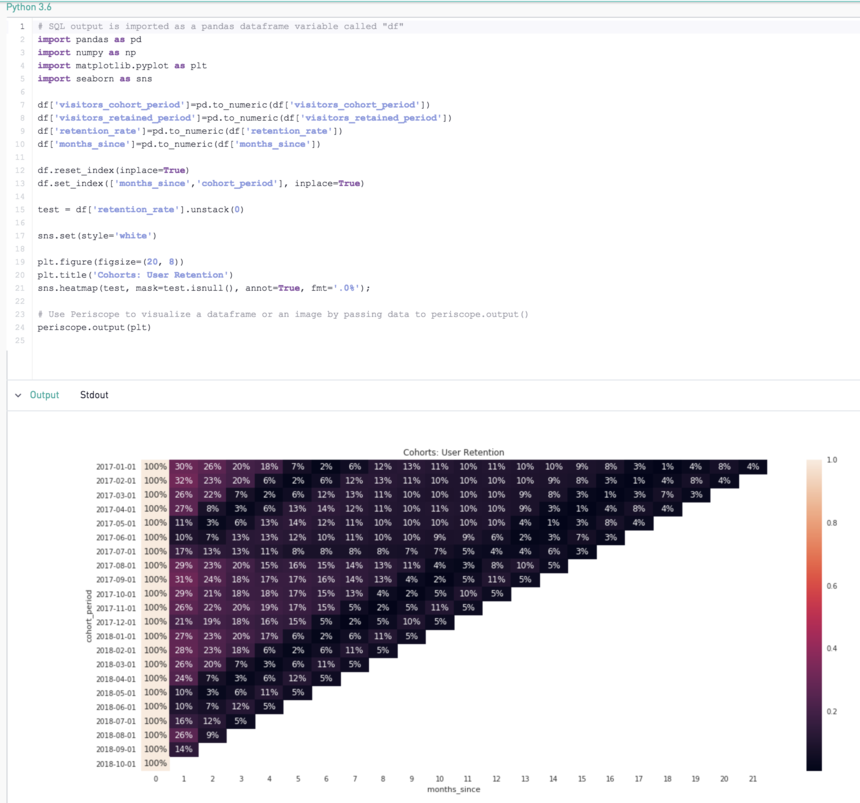cohort analysis grid visualization with Python, Pandas, matplotlib and Seaborn

I want to share some code I put together to make a cohort grid using Python, Pandas, matplotlib and Seaborn in case it's useful to others.

# SQL output is imported as a pandas dataframe variable called "df"
import pandas as pd
import numpy as np
import matplotlib.pyplot as plt
import seaborn as sns

df['visitors_cohort_period']=pd.to_numeric(df['visitors_cohort_period'])
df['visitors_retained_period']=pd.to_numeric(df['visitors_retained_period'])
df['retention_rate']=pd.to_numeric(df['retention_rate'])
df['months_since']=pd.to_numeric(df['months_since'])

df.reset_index(inplace=True)
df.set_index(['months_since','cohort_period'], inplace=True)

test = df['retention_rate'].unstack(0)

sns.set(style='white')

plt.figure(figsize=(20, 8))
plt.title('Cohorts: User Retention')
sns.heatmap(test, mask=test.isnull(), annot=True, fmt='.0%');

# Use Periscope to visualize a dataframe or an image by passing data to periscope.output()
periscope.output(plt)

The visualization ended up looking like this:Also thanks to Neha for her help debugging a few data type issues!

Sources:

2replies Oldest first
• Oldest first